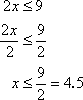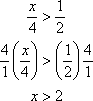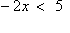Solving Linear Inequalities:
Elementary Examples
(page 2 of 3)

Sections: Introduction and formatting, Elementary examples, Advanced examples• Solve2x < 9.

If they had given me "2x = 9", I would have divided the 2 from each side. I can do the same thing here:Then the solution is: x < 9/2

...or, if you prefer decimals (and if your instructor will accept decimal equivalents instead of fractions):

Advertisement

x < 4.5

• Solve x/4 > 1/2.

If they had given me " x/4 = 1/2 ", I would have multiplied both sides by 4. I can do the same thing here:Then the solution is: x > 2

• Solve –2x < 5.

Remember how I said that solving linear inequalities is "almost" exactly like solving linear equations? Well, this is the one place where it's different. To explain what I'm about to do, consider the following:   Copyright © Elizabeth Stapel 2002-2011 All Rights Reserved

3 > 2

What happens to the above inequality when I multiply through by –1? The temptation is to say that the answer will be "–3 > –2". But –3 is not greater than –2; it is in actuality smaller. That is, the correct inequality is actually the following:

–3 < –2

As you can see, multiplying by a negative ("–1", in this case) flipped the inequality sign from "greater than" to "less than". This is the new wrinkle for solving inequalities:

When solving inequalities, if you multiply or divide through by a negative, you must also flip the inequality sign.

To solve "–2x < 5", I need to divide through by a negative ("–2"), so I will need to flip the inequality:Then the solution is: x > –5/2

• Solve (2x – 3)/4  < 2.

First, I'll multiply through by 4. Since the "4" is positive, I don't have to flip the inequality sign:

(2x – 3)/4   < 2
(4) × (2x – 3)/4  < (4)(2)

2x – 3 < 8

2x < 11

x < 11/2  = 5.5

You can use the Mathway widget below to practice solving a linear inequality. Try the entered exercise, or type in your own exercise. Then click the "paper-airplane" button to compare your answer to Mathway's. (Or skip the widget and continue with the lesson.)

Please accept "preferences" cookies in order to enable this widget.

(Clicking on "Tap to view steps" on the widget's answer screen will take you to the Mathway site for a paid upgrade.)

• Solve 10 < 3x + 4 < 19.

This is what is called a "compound inequality". It works just like regular inequalities, except that it has three "sides". So, for instance, when I go to subtract the 4, I will have to subtract it from all three "sides".

10 < 3x + 4 < 19
6 < 3x < 15

2 < x < 5

You can use the Mathway widget below to practice solving an interval inequality. Try the entered exercise, or type in your own exercise. Then click "the "paper-airplane" button to compare your answer to Mathway's. (Or skip the widget and continue with the lesson.)

Please accept "preferences" cookies in order to enable this widget.

(Clicking on "Tap to view steps" on the widget's answer screen will take you to the Mathway site for a paid upgrade.)

<< Previous  Top  |  1 | 2 | 3  |  Return to Index  Next >>

 Cite this article as: Stapel, Elizabeth. "Solving Linear Inequalities: Elementary Examples." Purplemath. Available from     https://www.purplemath.com/modules/ineqlin2.htm. Accessed [Date] [Month] 2016

MathHelp.com Courses
 Copyright © 2021  Elizabeth Stapel   |   About   |   Terms of Use   |   Linking   |   Site Licensing Contact Us